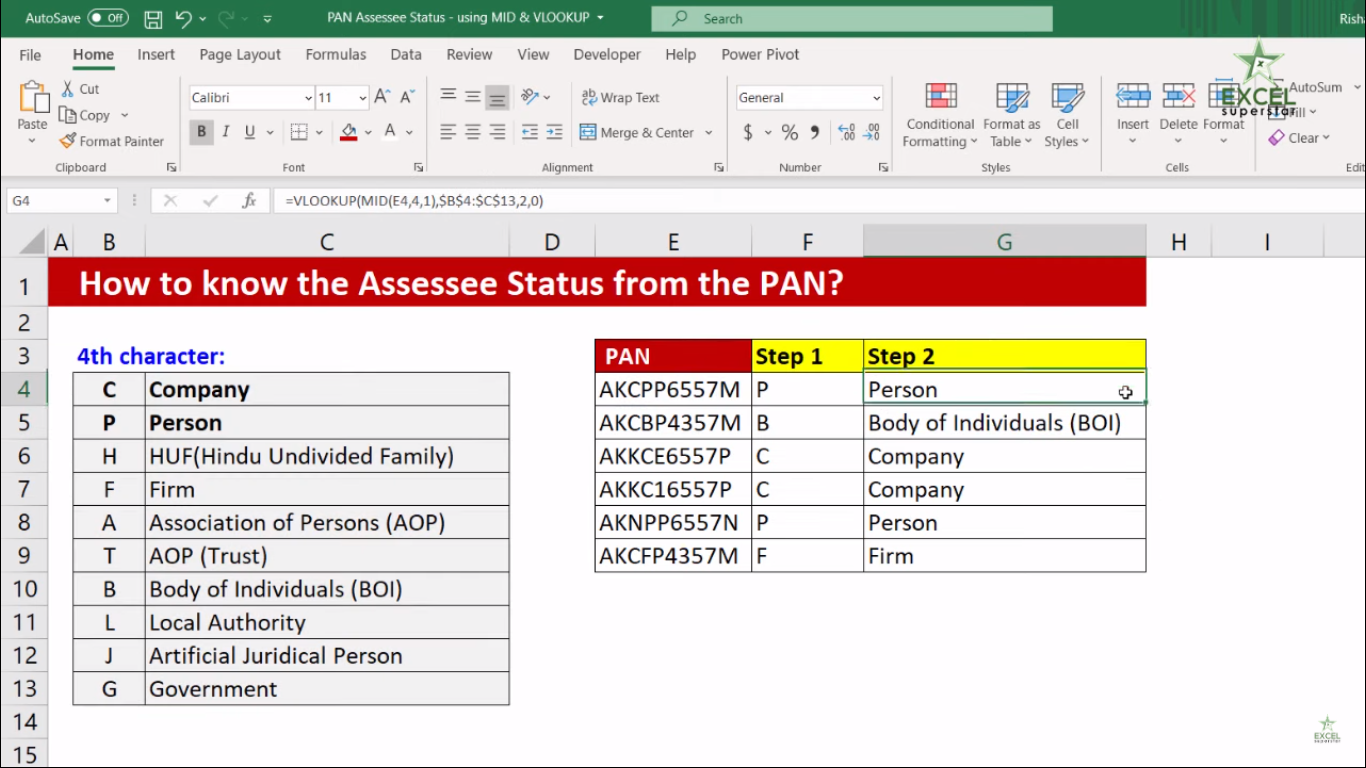# Assessee status from Pan Card using ExcelIn this tutorial we will learn about how to know the Assessee status from Pan Card using Excel Formulas with the help of an example given below: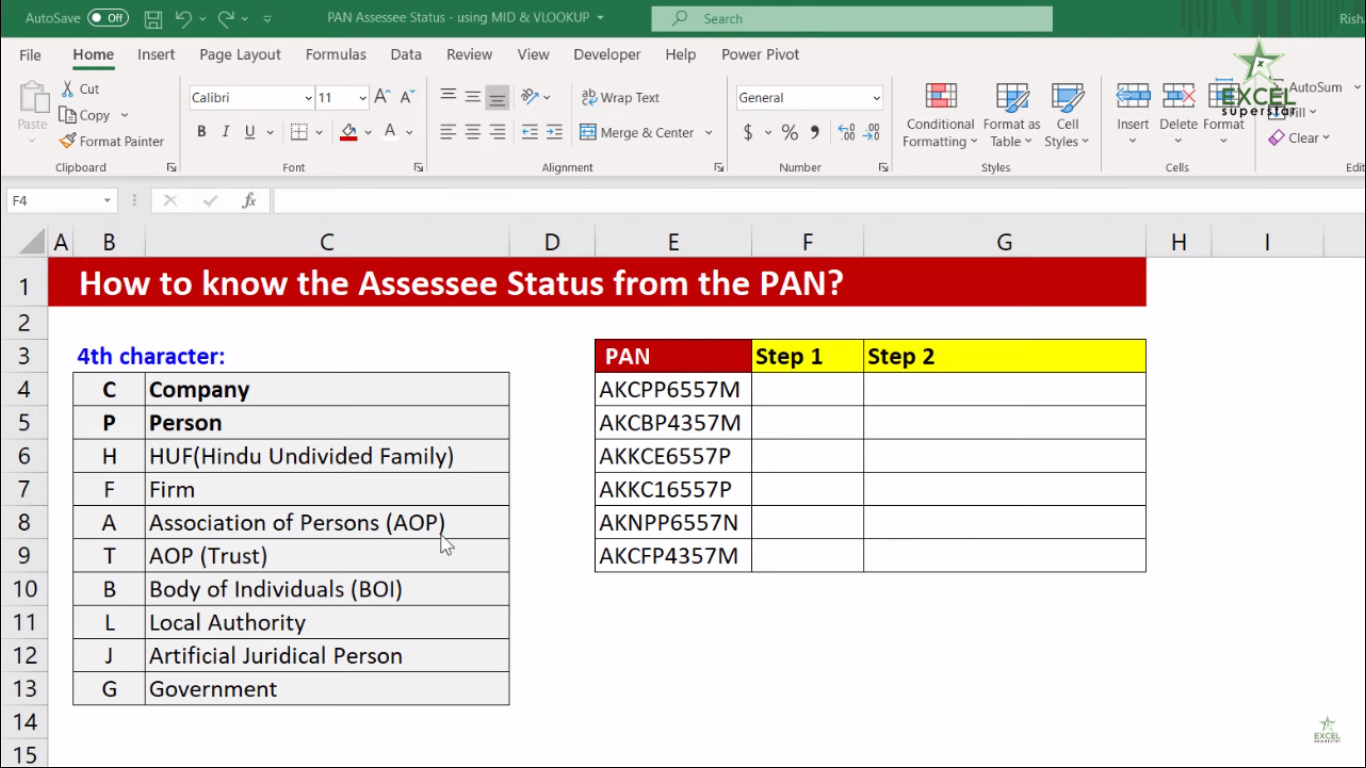Now here I have a table along with the Pan Card Numbers given in each row and, along with that, there is a table named as 4th Character along with their short forms.

So now, How can I know the Assesses (4th Character) from Pan Card using Excel from the table?

Note – The Fourth Character in the Pan Number indicates whether you are a company, person, Body of Individuals or Government Entity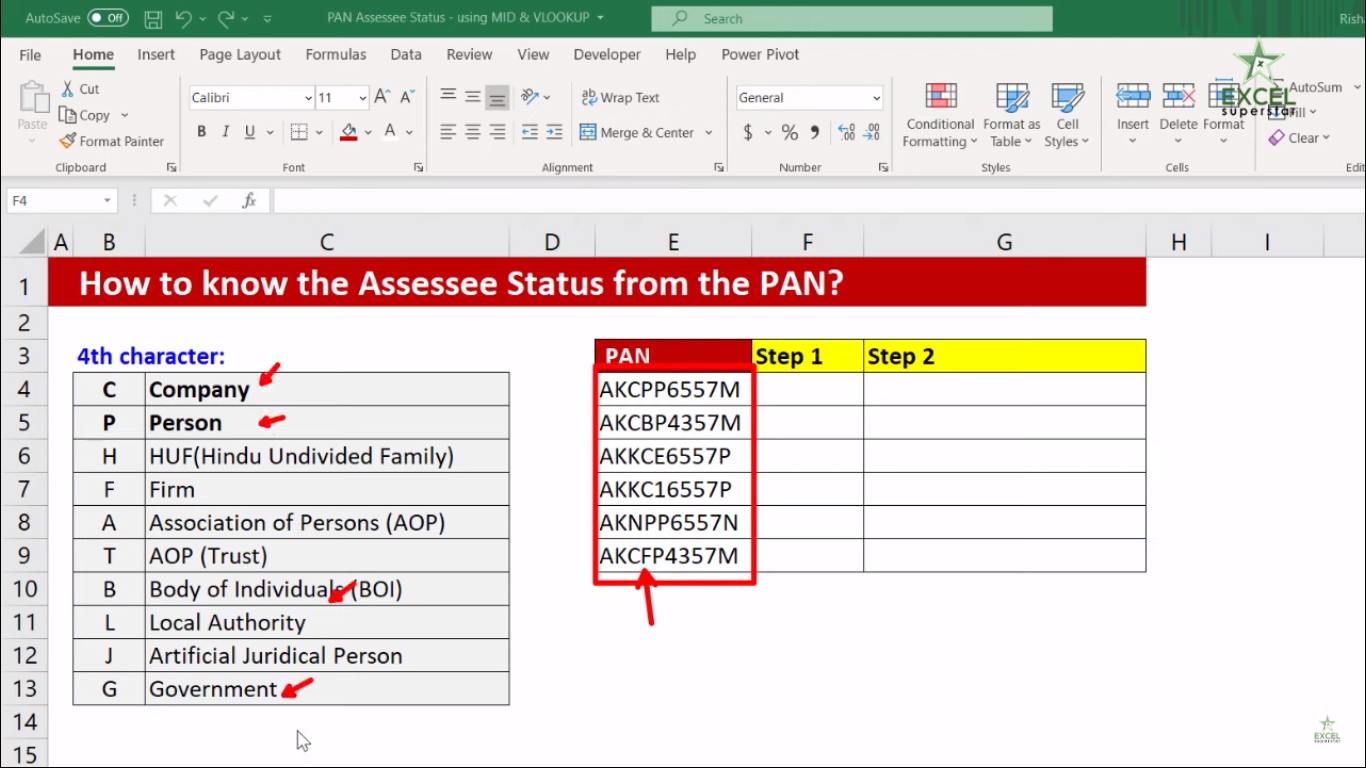To know the Assesses status from Pan Card using Excel we will be applying 2 steps

Step 1. MID Formula

Step 2. VLOOKUP Formula

Step 1. MID Formula

1. Press =MID and open the bracket =MID(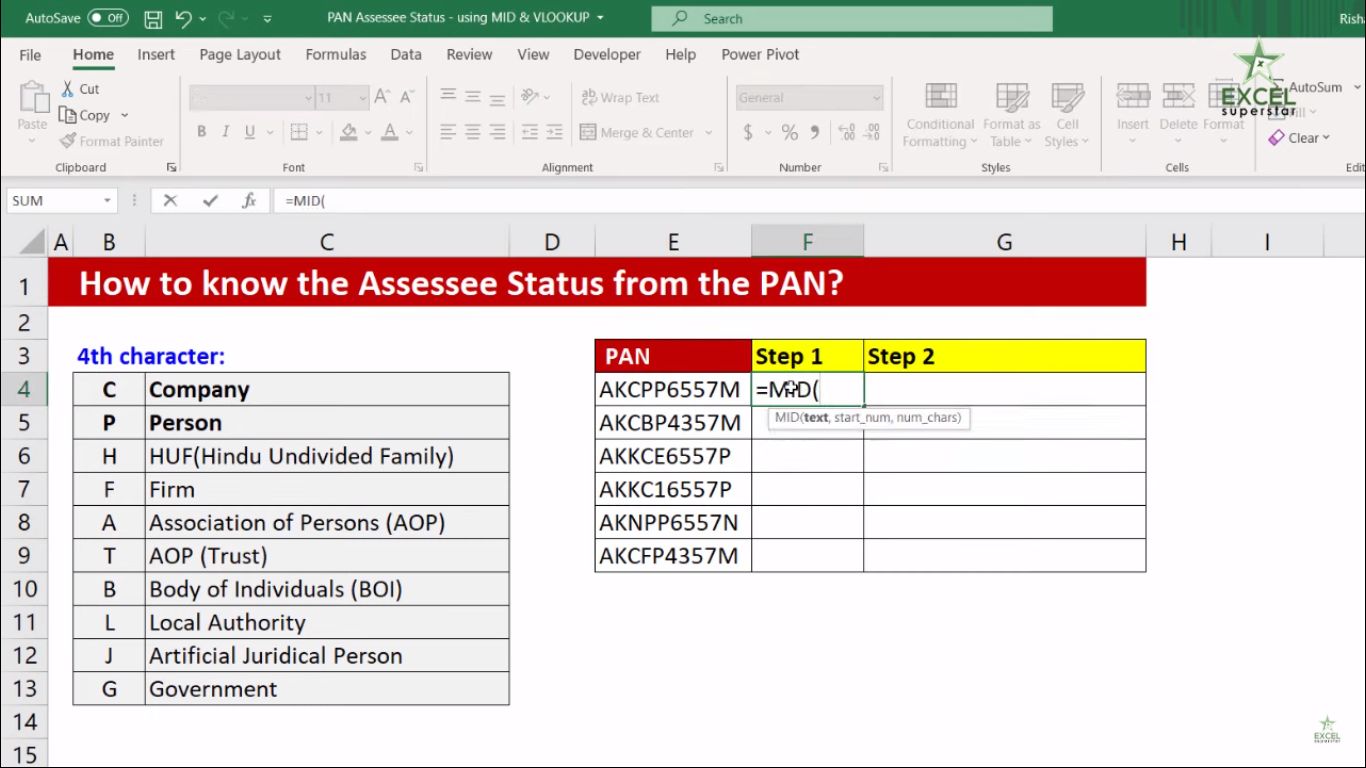Now here there are 3 parameters (text, start_num , num_chars).

The first parameter text means text from which you want to extract the substring.

2. Choose the cell where the Pan Number is written and press comma (,) =MID(E4,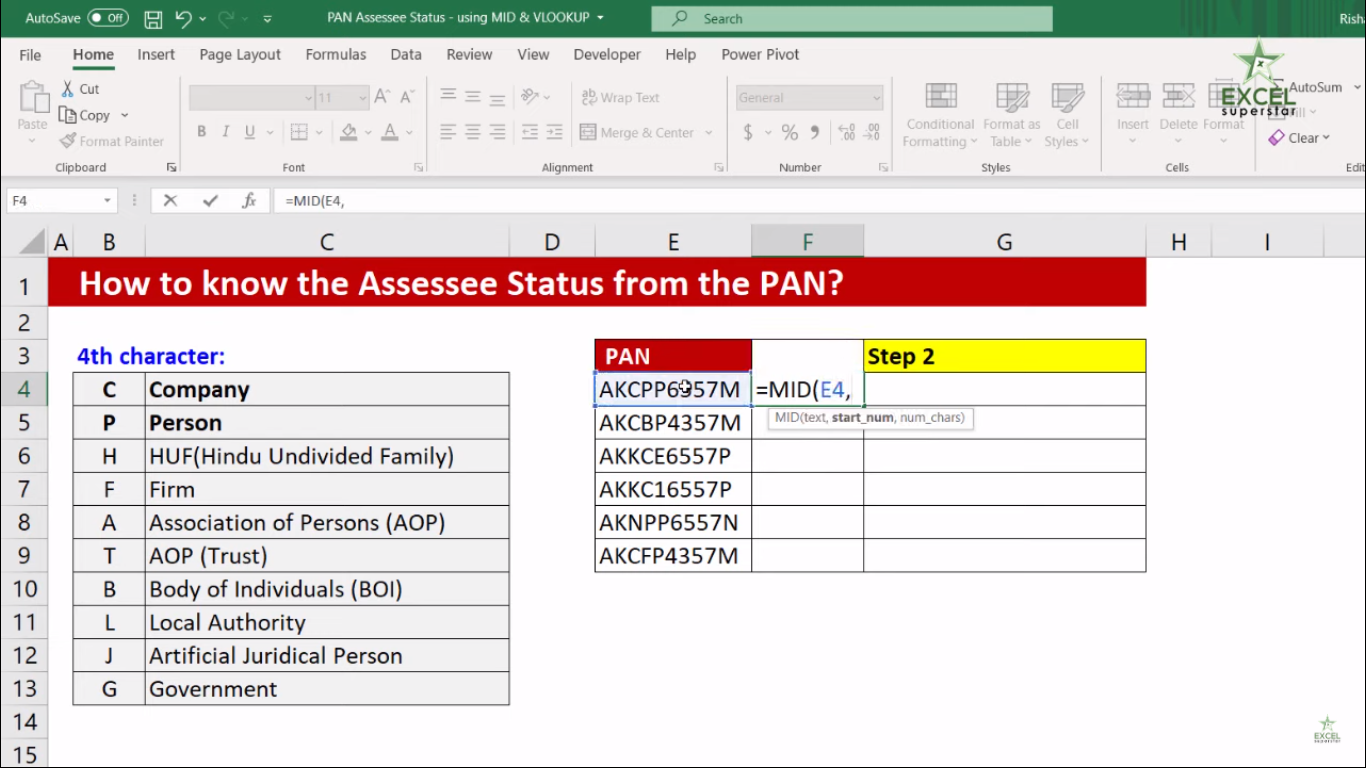The second parameter start_num means the starting position from which you want to execute your data

3. So write 4 and press comma =MID(E4,4,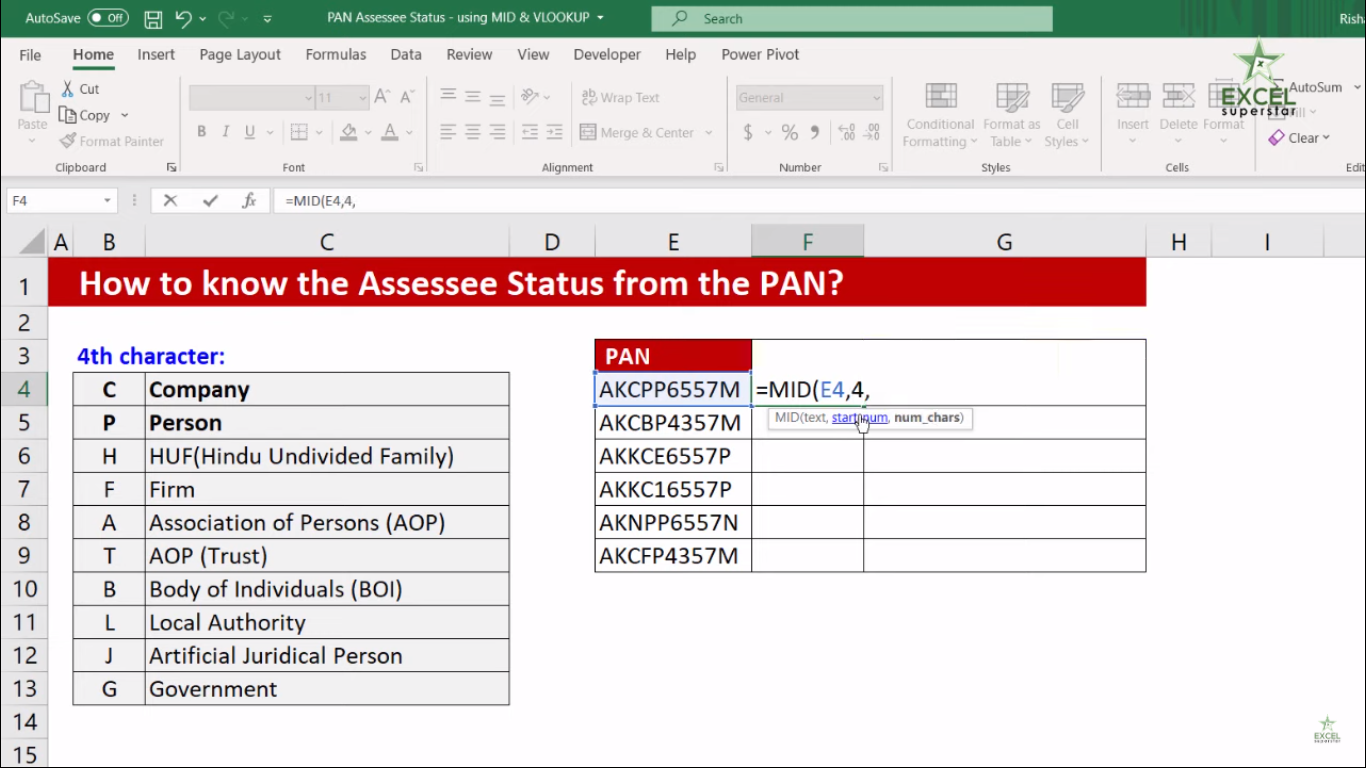The third parameter num_chars means the number of characters which you want to execute from your data

4. So write 1 as a character which you want to execute and close the bracket =MID(E4,4,1)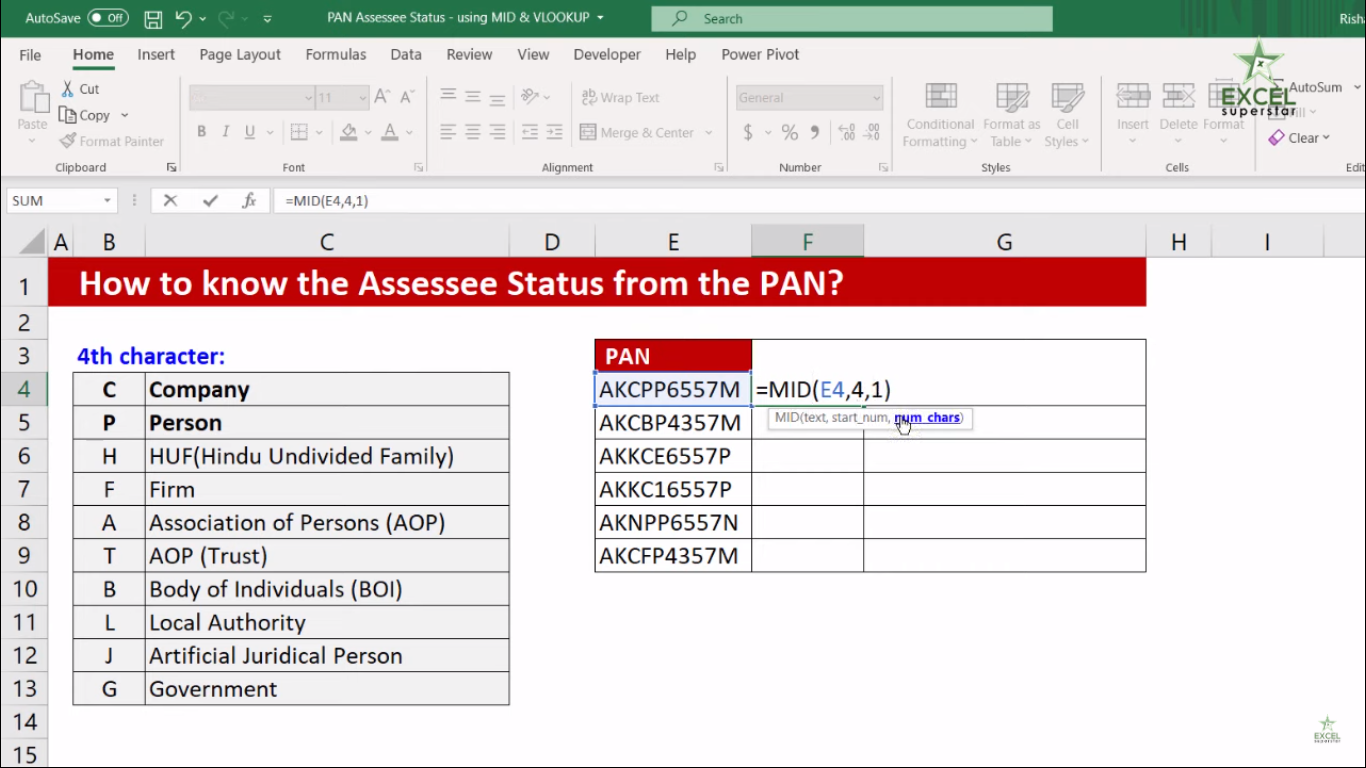5. Press Enter

6. Copy the Pan Number and drag it to the bottom of the table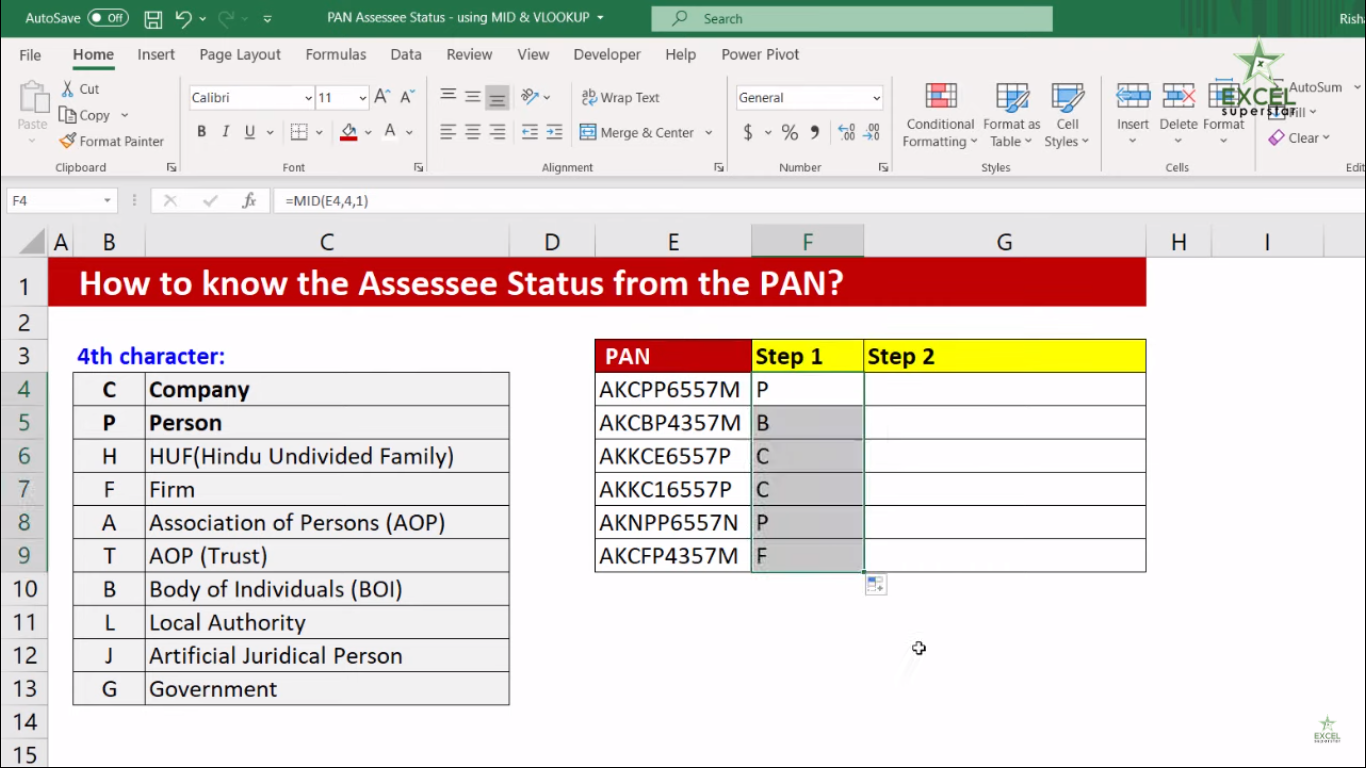Now the 4th Character is been executed from the Pan Card Number so the next step is to apply VLOOKUP Formula

Step 2: VLOOKUP Formula

1. Press =VLOOKUP and open the bracket =VLOOKUP(Now there are 4 parameters (lookup_value, table_array, col_index_num , [range_lookup]).

The first parameter lookup_value means the value to search for in the first column of the table.

2. Choose the 4th Character cell which is executed from the MID Formula =VLOOKUP(F4,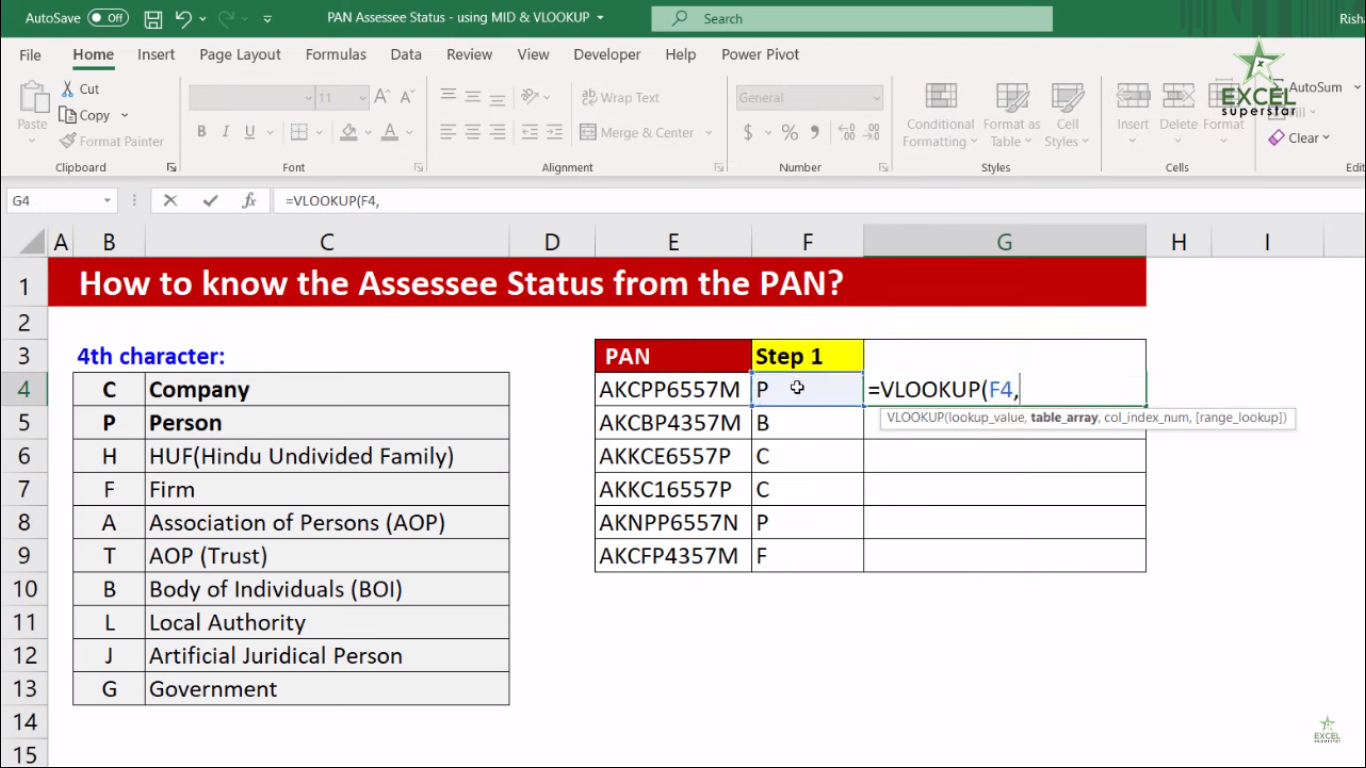The second parameter Table_array means two or more columns of data which is sorted in ascending order.

3. Select the entire 4th Character Table and press F4 Key =VLOOKUP(F4, \$B\$4:\$C\$13,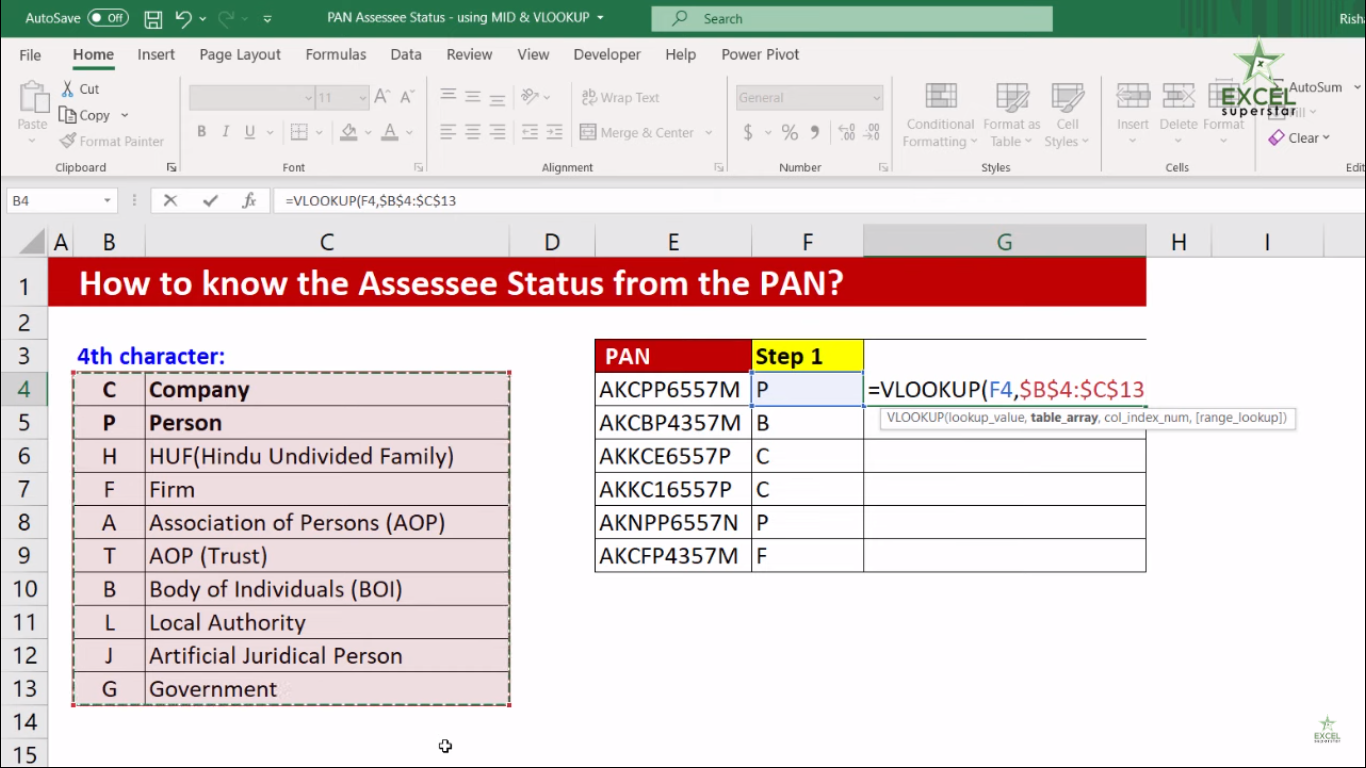The third parameter col_index_num means column number in table from which the matching value must be returned

4. Then press 2 as column number =VLOOKUP(F4, \$B\$4:\$C\$13,2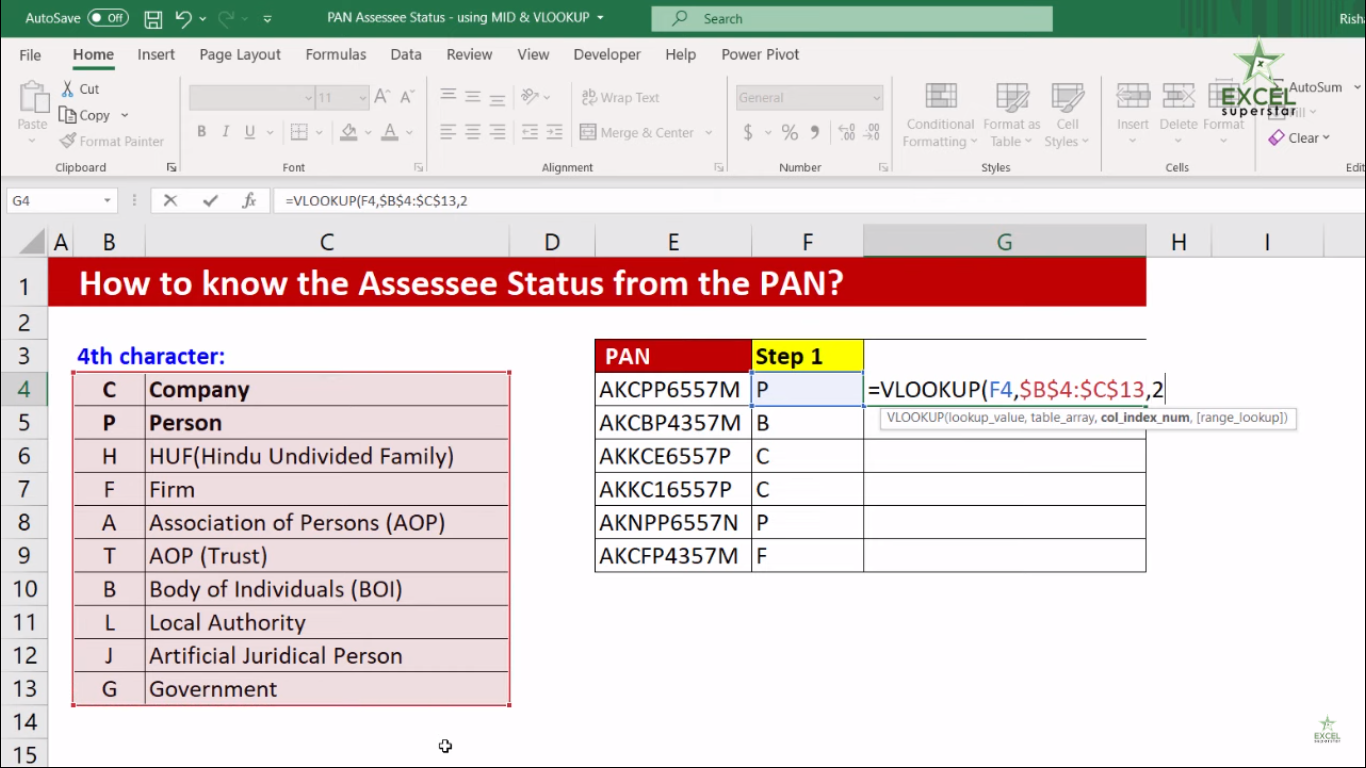The fourth character [range_lookup] means TRUE or FALSE for a match

5. So write 0 or FALSE for exact match and close the bracket =VLOOKUP(F4, \$B\$4:\$C\$13,2,0)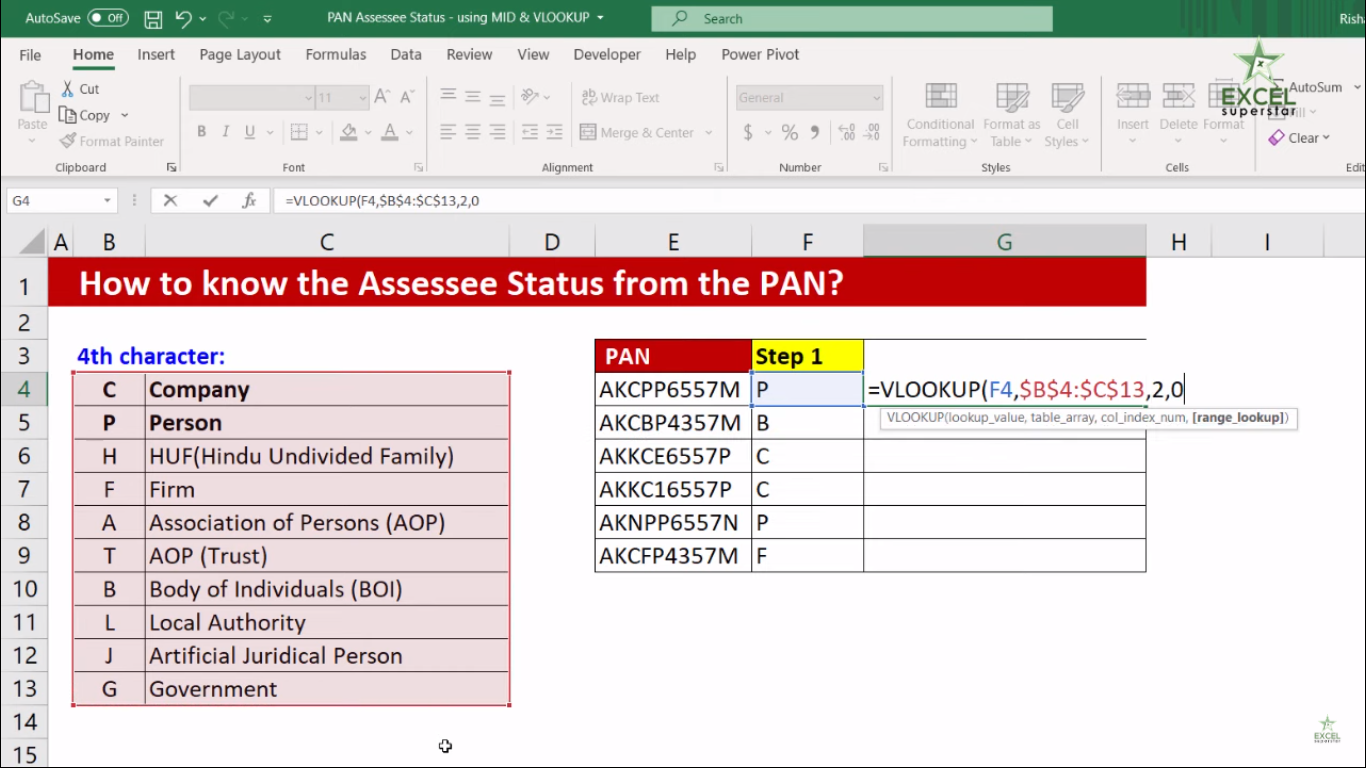6. Press Enter

7. Copy the formula and drag it to the bottom of the table and you will see the Assesses in the Pan Card Number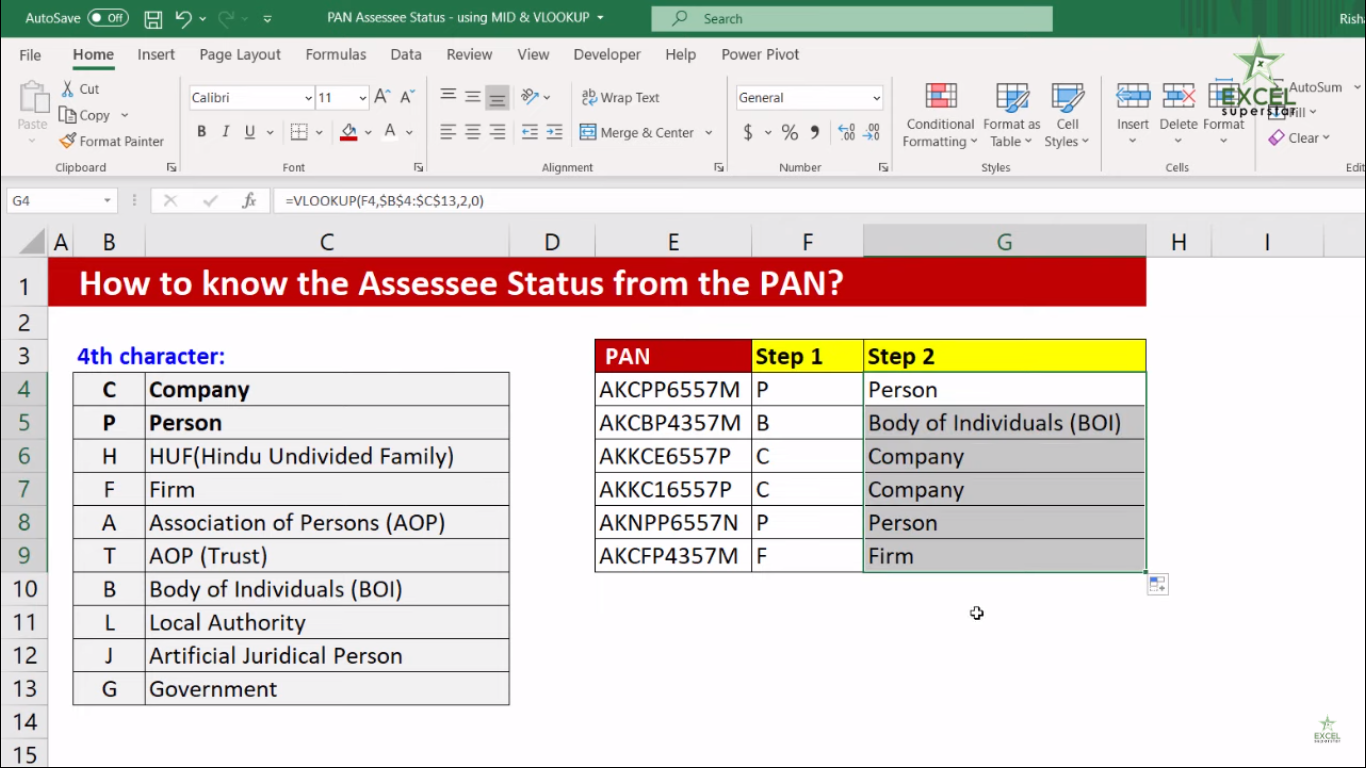You can also apply the MID Formula inside the VLOOKUP Formula. So follow the steps given below to do it:

1. Copy the MID Formula without equal to (=) sign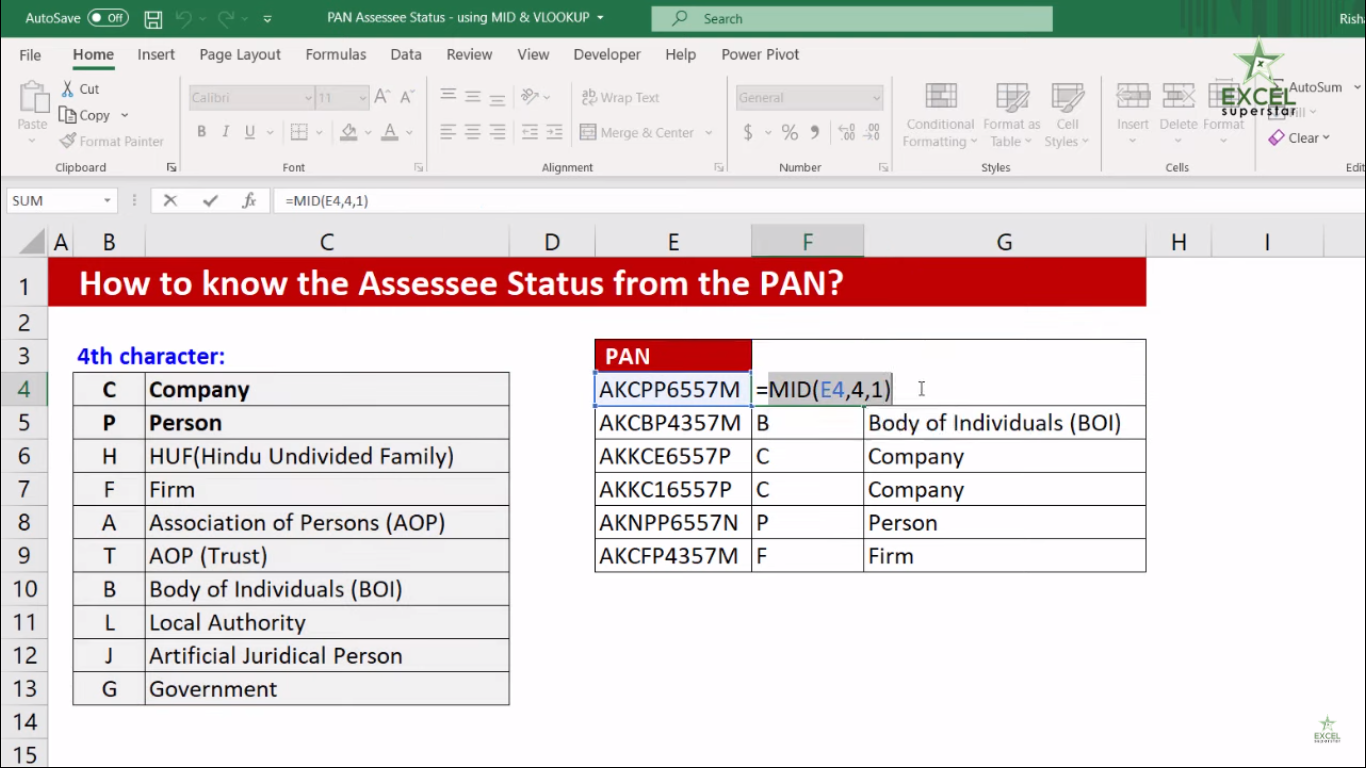2. Go to VLOOKUP Formula and replace the lookup_value parameter to MID Formula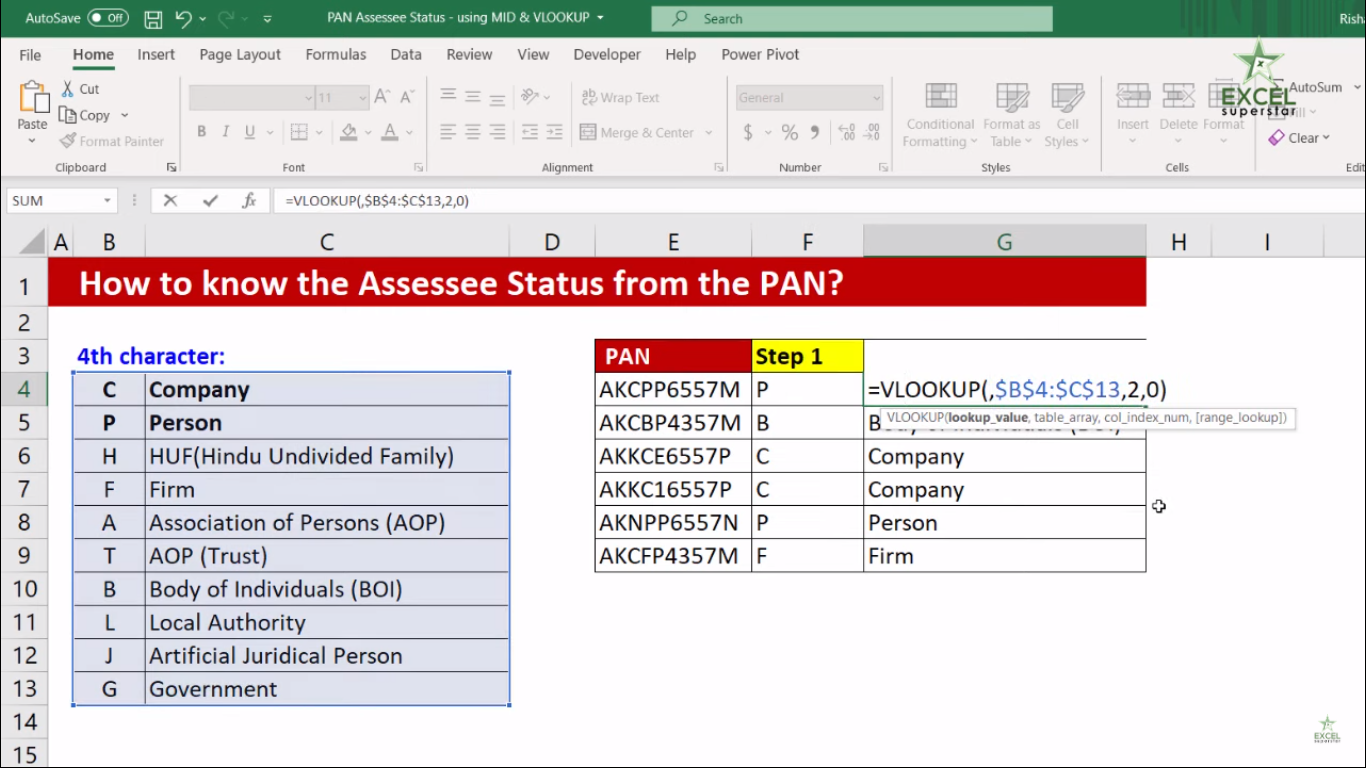4. Press Enter and you will see a single consolidated formula which tells you how to know the Assesses status from Pan Card